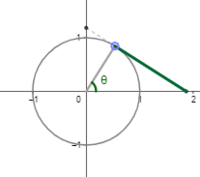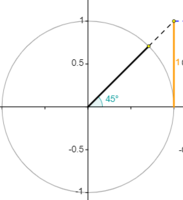# Tan graph from unit circle..

#### apple2357

##### Full Member
I have seen a couple of ways of developing the Tan graph from the unit circle.
One is this diagram:with a point on the circumference moving and the length of the green line being measured.

Another is this one:where a point is on the line x=1

How are these connected? And which do you favour as a way of developing the tan graph? I had only met the first one before and the second is fairly new to me?

#### lev888

##### Senior Member
Do you understand how they work? If you do, then you know how they are connected.

#### Jomo

##### Elite Member
I have never seen either before. Very interesting.

In the 1st one you say that the green line is measured. Are you saying that its length is changing as the point on the circumference moves?

I suspect not (in that case the length of the green line is sqrt(3)). In that case the 2nd one follows from just rotating the circle so you get the same figure as the 1st one (the difference will be that the green line and the yellow line will be difference lengths but we can fix that).

#### lev888

##### Senior Member
Are you saying that its length is changing as the point on the circumference moves?
It changes - the line needs to remain tangent. We want a right triangle.
These are 2 ways of getting a triangle with one leg of size 1, so the tangent is the size of the other leg.

#### Dr.Peterson

##### Elite Member
I have seen a couple of ways of developing the Tan graph from the unit circle.
One is this diagram:

View attachment 17410

with a point on the circumference moving and the length of the green line being measured.

Another is this one:

View attachment 17411

where a point is on the line x=1

How are these connected? And which do you favour as a way of developing the tan graph? I had only met the first one before and the second is fairly new to me?
I think the second one is easier to connect to the graph, as you just have to watch the point at the top of the yellow line move as the radial line rotates.

In each case, the idea is that the colored line is the "opposite" side and the "adjacent" side is 1, so the colored line is the tangent.

In this picture, you can see that the segment marked tan and segment AE are clearly equal.

#### Otis

##### Senior Member
I have never seen either before …
That surprises me somewhat, Jomo. All six trig functions may be represented by line segments on such a graph. Be sure to check out the link, in post #5. Those interpretations are interesting.#### apple2357

##### Full Member
I have never seen either before. Very interesting.

In the 1st one you say that the green line is measured. Are you saying that its length is changing as the point on the circumference moves?

I suspect not (in that case the length of the green line is sqrt(3)). In that case the 2nd one follows from just rotating the circle so you get the same figure as the 1st one (the difference will be that the green line and the yellow line will be difference lengths but we can fix that).
Sorry should have been clearer, the green line is a segment from a point on the unit circle to the x axis

#### apple2357

##### Full Member
I think the second one is easier to connect to the graph, as you just have to watch the point at the top of the yellow line move as the radial line rotates.

In each case, the idea is that the colored line is the "opposite" side and the "adjacent" side is 1, so the colored line is the tangent.

In this picture, you can see that the segment marked tan and segment AE are clearly equal.
Clearly equal? Am i missing something?
Congruent triangles?

Last edited:

#### Otis

##### Senior Member
Clearly equal? Am i missing something?
Hi apple. Yes and yes. (I mean, it's clear with a little mental effort.)

The blue segment and the segment AE are each a side of a right triangle. Both of those triangles have a leg measuring 1 unit, and both contain the same acute angle (theta). Hence, they are the same triangle.#### apple2357

##### Full Member
Hi apple. Yes and yes. (I mean, it's clear with a little mental effort.)

The blue segment and the segment AE are each a side of a right triangle. Both of those triangles have a leg measuring 1 unit, and both contain the same acute angle (theta). Hence, they are the same triangle.yes i agree! It was obvious once i stripped away the other stuff going on!